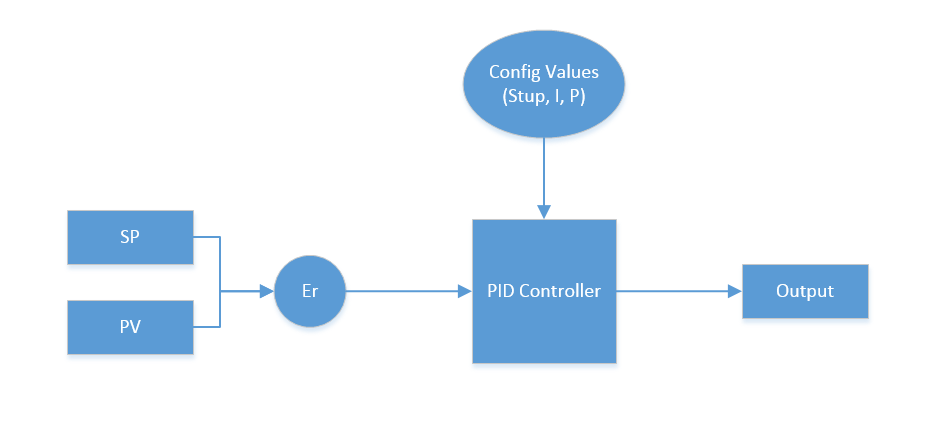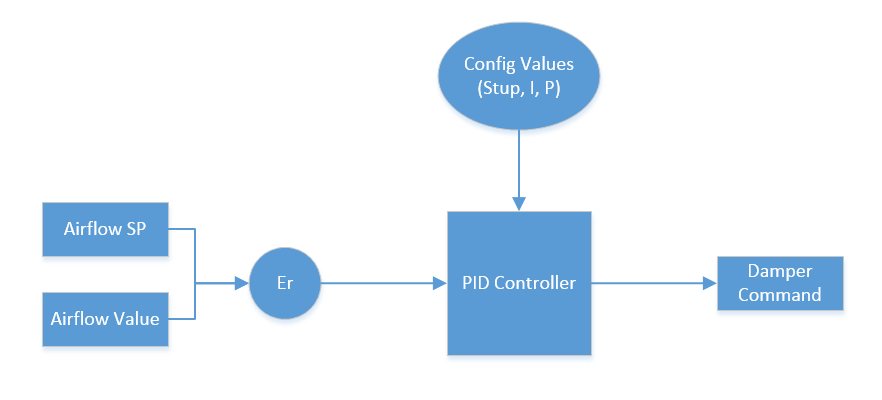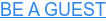[smart_track_player url="http://traffic.libsyn.com/buildingautomationmonthly/Episode17.mp3" title="BAM Episode 017: The How, What and Why of PID Loops" social="true" social_twitter="true" social_facebook="true" social_gplus="true" social_linkedin="true" social_email="true" hashtag="smartbuildings" ]

Welcome to the first ever Learn Series article!

In today's article I will be discussing PID loops.

Specific to this topic I will be teaching you:

• What are PID Loops?
• How do PID Loops function?

So why does this matter to you? Well folks PID loops are the backbone of building automation systems.

There's no easy analogy to give you around PID loops as they really are a unique feature of control systems. But there is good news, while PID loops may be unique they can be easily explained.

## What are PID Loops?

Proportional Integral Derivative (PID) loops are the main process loop used to control building automation systems. Got that? Good, we're done here.

Seriously though, does that explanation confuse the crap out of you? It did for me!

Most of the stuff written about PID loops is confusing, but it doesn't have to be.

Here's the deal. PID loops simply adjust the command sent to an output based on the difference between a set-point and an input.

Got it?

Consider this scenario.

You have a room controller.

This room controller has an airflow set-point and an airflow sensor, which will be our process variable (PV) . The room controller also has a damper with an actuator on it that controls the airflow.

As the airflow gets further away from set-point the PID loop increases the output. Pretty easy right? Well that my friends is a PID loop!

## How do PID Loops Function?

But how does it really work?

Ok, you want the nitty-gritty details? Here it goes!

PID Loops have a couple of key terms:

• Process Variable (PV) - The input that is being measured and impacted by the output
• Set-point (SP) - The value that the input is supposed to be
• Error (Er) - The difference between the input and the process variable
• Proportional - A scaled representation of the error
• Integral (I) - The amount of time the PV and SP have been different
• Start-up Value (Stup) - The value the PID loop puts out when it first startsAlright, so when you set up a PID Loop in programming software you connect the Pv (Input) and Sp (Set-point) to the logic block.

Next you set the I and P gains. What are I and P gains you ask? Great question!

The difference between your set-point and input is called error. This error gets entered into the PID controller. The first thing this controller does is to determine the proportional impact of the error.

How does this happen? Well you have a tuning factor called the proportional gain often called (Kp). This is part of the tuning process that will be covered in next weeks article.

Suffice to say different processes have different gain settings. Think of this as setting the dead-band range for a conventional thermostat.

The next factor you have is the integral factor. This means time, basically you are determine how long you have been away from set-point. Now this is the simple explanation there's actually much more to this, with past error summation and other mathy topics...

However, for the purpose of this conversation simply understand the while the proportional value will be proportional to the error, the integral value will gradually grow over time. You control this increase by setting the Integral gain limit and Integral max values (Ilim) and (Imax).

Now all you have to do is to let the loop run.Let's look back at our previous scenario now that we know a bit more about how PID loops work. In our scenario, the difference between the airflow sensor (PV) and the air-flow set-point (SP) is the error.

This error value feeds into the PID loop where the PID loop then controls the output based on the P and I tuning factors.

## Conclusion

So why do we use PID loops? Um, because they work..

Seriously though, PID loops are only one of many forms of control sequences. In future articles we will discuss other control sequences. What I want to caution you on is PID loops are not a band-aid solution.

I've seen folks use PID loops to control systems that could have been controlled using other means.

In next weeks article we will discuss when to use PID loops and how to tune PID loops.

Let me know your thoughts in the comment section below.### Want to be a guest on the Podcast?